Chapter Notes: Coordinate Geometry

# Coordinate Geometry Chapter Notes - Mathematics (Maths) Class 9

 Table of contentsIntroduction to Coordinate GeometryCartesian SystemCoordinates of a Point in Cartesian PlaneSigns of Coordinates in different Quadrants## Introduction to Coordinate Geometry

Suppose I put a small dot on a sheet of paper with a pen. Can you locate this dot on the paper if I tell you that the dot is at the lower right corner of the paper?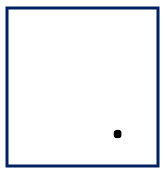Now, you are able to see the dot but, can you tell me the exact position of the dot?
You will see that the information given above is not sufficient to fix the position of the dot.

Now, if I tell you that the point is nearly 2 cm away from the bottom line of the paper then this will give some idea but still is not sufficient because this would mean that the point could be anywhere, which is 2 cm away from the bottom line.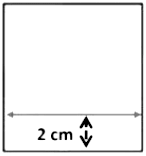Therefore, to fix the position of the dot we have to specify its distance from two fixed lines,  the right edge and the bottom line of the paper. Therefore, if I say that the dot is also 1 cm away from the right edge of the paper, then we can easily fix the position of the dot.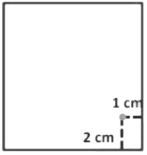We see that position of any object lying in a plane can be represented with the help of two perpendicular lines.
Coordinate geometry is the branch of mathematics where we study the position of an object on a plane with reference to two mutually perpendicular lines in the same plane.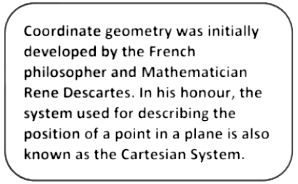## Cartesian System

### Number Line

• The number line is used to represent the numbers by marking points on a line at equal distances.
• On a number line distances from the fixed point are marked in equal units positively in one direction and negatively in the other.
• This fixed point from which the distances are marked is called the origin. In the figure 0 denotes the origin.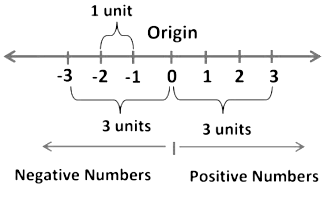3 on number line is located at a distance of 3 units on the right side of origin 0. Similarly, -3 is located at the same distance from origin but on its left side.

In Cartesian system, two perpendicular lines are used, one of them is horizontal (XX’) and the other is vertical (YY’).

• The horizontal line X'X is called the x — axis and the vertical line Y'Y is called the y — axis.
• The point where X'X and Y'Y intersect is called the origin (denoted by O).
• Directions OX and OY are the positive directions of X - axis and Y - axis, respectively.
• Similarly, directions OX' and OY' are the negative directions of X -axis and Y - axis, respectively.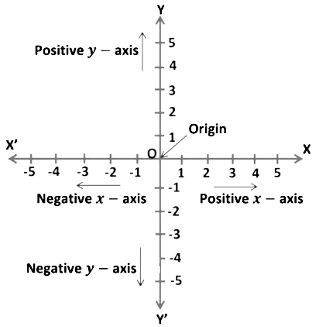The axes (plural of the word ‘axis’) divide the plane into four parts. These four parts are called the quadrants (1/4), numbered I, II, III and IV anticlockwise from OX.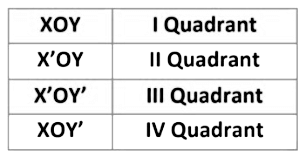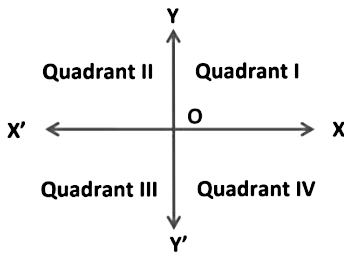The plane consists of the axes and the four quadrants. We call the plane, the Cartesian plane, or the coordinate plane, or the xy-plane. The axes are called the coordinate axes.
A plane is a flat surface that goes infinitely in both directions

Question for Chapter Notes: Coordinate Geometry
Try yourself:Which statement is true about the number line?

##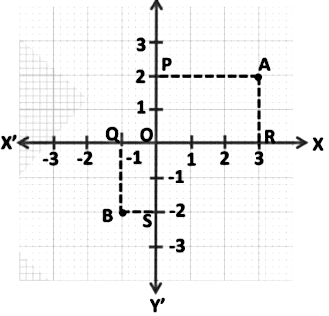• The perpendicular distance of a point from the y axis measured along the x axis is called its x coordinate, or abscissa. For the point A it is +3 and for B, it is -1.
• The perpendicular distance of a point from the x axis measured along the y axis is called its y coordinate, or ordinate. For the point A it is +2 and for B, it is -2.
• In stating the coordinates of a point in the coordinate plane, the x -coordinate comes first, and then the y - coordinate. We place the coordinates in brackets. Therefore, coordinates of A are (3,2) and B are (-1, -2).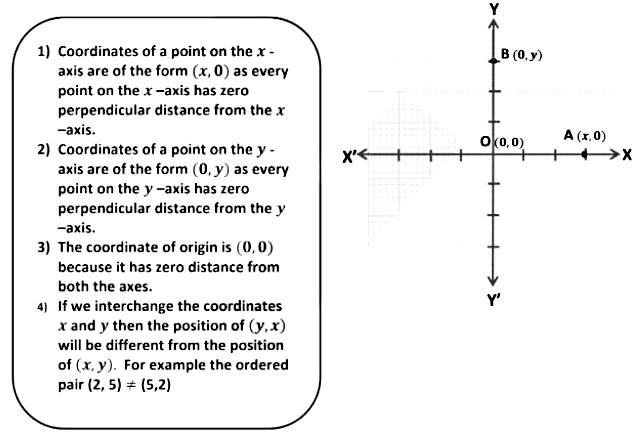Example: Point P is on the x-axis and is at a distance of 3 units from the y-axis to its left. Write the coordinates of point P.

Point P is at a distance of 3 units towards left, from  y-axis.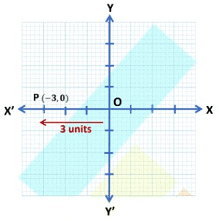Coordinates of point P are (-3, 0).

Example: Find distances of points C (-3, -2) and D (2, 1) from x-axis and y-axis.

C (-3, -2)
Distance from x − axis = 2 units
Distance from y − axis = 3 units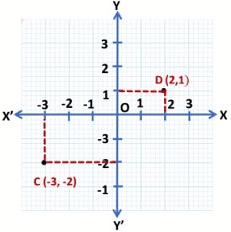D (2, 1)
Distance from x − axis = 1 units
Distance from y-axis = 2 unit

Example: Locate and write the coordinates of a point:
(a) lying on the x-axis to the left of origin at a distance of 4 units. b) above x-axis lying on the y-axis at a distance of 4 units from the origin.
b) above x- axis lying on y- axis at a distance of 4 units from origin.

(a) The given point is at a distance of 4 units towards left from the y-axis and at a zero distance from the x-axis. Therefore, the x − coordinate of the point is -4 and the y − coordinate is 0.
Hence, the coordinates of the given point are (-4, 0). Coordinates of a point on the x-axis are of the form (x, 0) as every point on the x-axis has zero perpendicular distance from the x-axis.
(b) The given point is at a zero distance from the y-axis at a distance of 4 units from the x-axis. Therefore, the x − coordinate of the point is 0 and the y − coordinate is 4. Hence, the coordinates of the given point are (0, -4). Coordinates of a point on the y-axis are of the form (0, y) as every point on the y-axis has zero perpendicular distance from the y-axis.

## Signs of Coordinates in different Quadrants

Example: Write the quadrant in which each of the following points lie:
(i) (-2, -4)
(ii) (1, -4)
(iii) (-3, 2)

(i) (-2, -4)

Here, x coordinate = -2 and y coordinate = -4
As x coordinate and y coordinate both are negative (x < 0, y < 0) ,the given point lies in III quadrant.

(ii) (1, -4)

Here, x coordinate = 1 and y coordinate = -4
As x coordinate is positive and y coordinate is negative (x > 0, y < 0 ) the given point lies in IV quadrant.

(iii) (-3, 2)

Here, x coordinate = -3 and y coordinate = 2
As x coordinate is negative and y coordinate is positive (x < 0, y < 0 ) the given point lies in II quadrant.

Example: If the coordinates of a point M are (-2, 9) which can also be expressed as (1 + x, y2) and y > 0, then find in which quadrant do the following points lie: P(y, x), Q(2, x), R(x2, y − 1), S(2x,−3y)

We know,
(-2, 9) = (1 + x , y2)
∴ -2 = 1 + x ⇒ x = -2 – 1
x = -3
9 = y2 ⇒ y = ± 3
Now, it is given that y > 0, so we choose the positive value of y.
So, y = 3
Therefore, x = -3 and y = 3
(i) P (y,  x)
P (y, x) = P (3, -3) (∵ y = 3 and x = -3)
As x coordinate is positive and y coordinate is negative (x > 0, y < 0 ) the given point lies in IV quadrant.
(ii) Q (2, x)
Q (2, x) = Q (2, -3) (∵ x = -3)
The x coordinate is positive and y coordinate is negative (x > 0, y < 0 ) so the given point lies in IV quadrant.
(iii) R (x2, y −1)
x= (−3)2 = 9; y −1 = 3 – 1 = 2
R (x2, y −1) = (9, 2)
As x coordinate and y coordinate both are positive (x > 0, y > 0) ,the given point lies in I quadrant.
(iv) S (2x, −3y)
2x =2 × (-3) = -6; -3y = -3 × 3 = -9
S (2x, −3y)= S (-6, -9)
As x coordinate and y coordinate both are negative (x < 0, y < 0),the given point lies in III quadrant.

Question for Chapter Notes: Coordinate Geometry
Try yourself:n the Cartesian coordinate system, which of the following points lies in the first quadrant?

The document Coordinate Geometry Chapter Notes | Mathematics (Maths) Class 9 is a part of the Class 9 Course Mathematics (Maths) Class 9.
All you need of Class 9 at this link: Class 9

## FAQs on Coordinate Geometry Chapter Notes - Mathematics (Maths) Class 9

 1. What is the Cartesian system?Ans. The Cartesian system, also known as the Cartesian coordinate system, is a mathematical system used to locate points in a two-dimensional plane. It uses two perpendicular lines, called axes, to determine the position of a point. The horizontal axis is called the x-axis, and the vertical axis is called the y-axis.
 2. How do you find the coordinates of a point in a Cartesian plane?Ans. To find the coordinates of a point in a Cartesian plane, we need to determine its distance from the x-axis and the y-axis. The distance from the x-axis is called the x-coordinate, and the distance from the y-axis is called the y-coordinate. The coordinates of a point are written as (x, y), where x represents the x-coordinate and y represents the y-coordinate.
 3. What are the signs of coordinates in different quadrants?Ans. In the Cartesian plane, the signs of coordinates vary in different quadrants. In the first quadrant, both the x-coordinate and y-coordinate are positive. In the second quadrant, the x-coordinate is negative, but the y-coordinate is positive. In the third quadrant, both the x-coordinate and y-coordinate are negative. In the fourth quadrant, the x-coordinate is positive, but the y-coordinate is negative.
 4. How do you plot coordinates on a Cartesian plane?Ans. To plot coordinates on a Cartesian plane, we start by locating the point where the x-coordinate and y-coordinate intersect. We then move horizontally (left or right) according to the value of the x-coordinate and vertically (up or down) according to the value of the y-coordinate. Finally, we mark the point where the horizontal and vertical lines intersect.
 5. What are some practical applications of coordinate geometry?Ans. Coordinate geometry has various practical applications in different fields. It is used in navigation systems to determine the location of an object. It is also used in computer graphics to create and manipulate images. In physics, coordinate geometry is used to study motion and calculate distances. Additionally, it is used in engineering, architecture, and surveying to design and measure structures.

## Mathematics (Maths) Class 9

62 videos|426 docs|102 tests

## Mathematics (Maths) Class 9

62 videos|426 docs|102 tests
Signup to see your scores go up within 7 days! Learn & Practice with 1000+ FREE Notes, Videos & Tests.
10M+ students study on EduRev
Track your progress, build streaks, highlight & save important lessons and more!(Scan QR code)
Related Searches

,

,

,

,

,

,

,

,

,

,

,

,

,

,

,

,

,

,

,

,

,

;